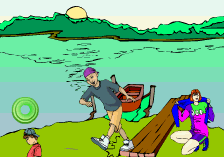Ch 2. Derivatives I Multimedia Engineering Math DerivativeFormulas TrigonometricFunctions Differentiability ChainRule ImplicitDifferentiation
 Chapter 1. Limits 2. Derivatives I 3. Derivatives II 4. Mean Value 5. Curve Sketching 6. Integrals 7. Inverse Functions 8. Integration Tech. 9. Integrate App. 10. Parametric Eqs. 11. Polar Coord. 12. Series Appendix Basic Math Units Search eBooks Dynamics Fluids Math Mechanics Statics Thermodynamics Author(s): Hengzhong Wen Chean Chin Ngo Meirong Huang Kurt Gramoll ©Kurt GramollMATHEMATICS - CASE STUDY IntroductionRate of increased area As Tom plays football with a group of friends he happens to kick the ball into some water. A girl nearby finds that the ball creates circular ripple that travele outward. She wonders how fast the circle area of the circular circles increase in size. What is known: The circular ripple travels outward at 2 ft/sec. Questions How fast does the circle of area increase after 3 seconds? Approach The rate of circular circles area change is the derivative of the area function.